Next: Square Potential Barrier Up: One-Dimensional Potentials Previous: Introduction

# Infinite Potential Well

Consider a particle of massand energymoving in the following simple potential: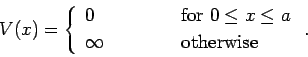(302)

It follows from Eq. (301) that if(and, hence,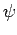) is to remain finite thenmust go to zero in regions where the potential is infinite. Hence,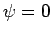in the regions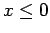and. Evidently, the problem is equivalent to that of a particle trapped in a one-dimensional box of length. The boundary conditions onin the regionare(303)

Furthermore, it follows from Eq. (301) thatsatisfies(304)

in this region, where(305)

Here, we are assuming that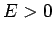. It is easily demonstrated that there are no solutions with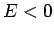which are capable of satisfying the boundary conditions (303).

The solution to Eq. (304), subject to the boundary conditions (303), is(306)

where the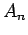are arbitrary (real) constants, and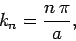(307)

for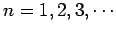. Now, it can be seen from Eqs. (305) and (307) that the energyis only allowed to take certain discrete values: i.e.,(308)

In other words, the eigenvalues of the energy operator are discrete. This is a general feature of bounded solutions: i.e., solutions in whichas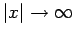. According to the discussion in Sect. 4.12, we expect the stationary eigenfunctions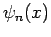to satisfy the orthonormality constraint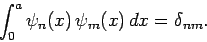(309)

It is easily demonstrated that this is the case, provided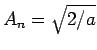. Hence,(310)

for.

Finally, again from Sect. 4.12, the general time-dependent solution can be written as a linear superposition of stationary solutions:(311)

where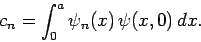(312)Next: Square Potential Barrier Up: One-Dimensional Potentials Previous: Introduction
Richard Fitzpatrick 2010-07-20